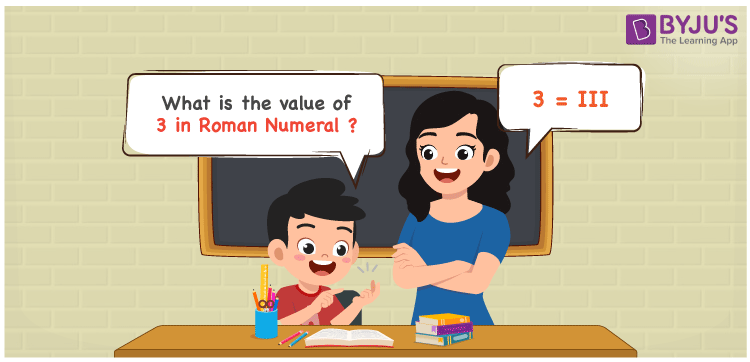# 3 in Roman Numerals

3 in Roman Numerals is III. There are seven symbols (alphabets) used in the Roman Numeral system. They are I, V, X , L, C, D and M. They represent the numbers 1, 3, 10, 30, 100, 300 and 1000 respectively. Since the number 3 is not a part of these 7 symbols, we represent the number 3 as a sum of 3 ones. For understanding the rules on Roman Numerals, you can refer to the link Rules to write Roman Numerals

 Number Roman Numeral 3 III

## How to Write 3 in Roman Numerals?3 does not have a primary symbol in the Roman numerals. So, 3 has to be broken down as 1 + 1 + 1. In the rules of Roman numerals, I can be repeated 3 times so, III will be 3.

Let us understand it Numerically;

3 = 1 + 1 + 1

3 = I + I + I

3 = III

## Video Lesson on Roman Numerals## Frequently Asked Questions on 3 in Roman Numerals

Q1

### How to write the number 3 in Roman Numerals?

3 in Roman Numerals is written as III.
Q2

### Does IIIII represent Roman Numerals?

No. IIII doesn’t represent Roman Numerals as it is invalid
Q3

### Can we represent 3 as the sum of 3 I’s in Roman numerals?

Yes. 3 is represented as the sum of 3 I’s in Roman numerals.